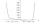# Equation

How many real roots has equation?

Result

n =  3

#### Solution:Leave us a comment of example and its solution (i.e. if it is still somewhat unclear...):Be the first to comment!#### To solve this verbal math problem are needed these knowledge from mathematics:

Do you have a linear equation or system of equations and looking for its solution? Or do you have quadratic equation?

## Next similar examples:

1. ChildrenThe group has 42 children. There are 4 more boys than girls. How many boys and girls are in the group?
2. Unknown numberI think number. If subtract from the twelfth square the ninth square I get a number 27 times greater than the intended number. What is this unknown number?55%+36%+88%+71%+100=63% what is whole (X)? Percents can be added directly together if they are taken from the same whole, which means they have the same base amount. .. . You would add the two percentages to find the total amount.
4. Fifth of the numberThe fifth of the number is by 24 less than that number. What is the number?
5. Three workshopsThere are 2743 people working in three workshops. In the second workshop works 140 people more than in the first and in third works 4.2 times more than the second one. How many people work in each workshop?
6. LegsCancer has 5 pairs of legs. The insect has 6 legs. 60 animals have a total of 500 legs. How much more are cancers than insects?
7. Elimination methodSolve system of linear equations by elimination method: 5/2x + 3/5y= 4/15 1/2x + 2/5y= 2/15
8. Three unknownsSolve the system of linear equations with three unknowns: A + B + C = 14 B - A - C = 4 2A - B + C = 0
9. ExaminationThe class is 21 students. How many ways can choose two to examination?
10. Geometric sequence 4It is given geometric sequence a3 = 7 and a12 = 3. Calculate s23 (= sum of the first 23 members of the sequence).
11. SequenceWrite the first 6 members of these sequence: a1 = 5 a2 = 7 an+2 = an+1 +2 an
12. PowerNumber ?. Find the value of x.
13. PowersExpress the expression ? as the n-th power of the base 10.
14. One halfOne half of ? is: ?
15. TrigonometryIs true equality? ?
16. Reference angleFind the reference angle of each angle:
17. Theorem proveWe want to prove the sentence: If the natural number n is divisible by six, then n is divisible by three. From what assumption we started?